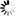DOI: 10.5176/2251-1911_CMCGS55

Authors: Homa Almasieh

Abstract: A direct method based on orthogonal triangular functions (TF)s is developed for solving the system of Fredholm integro-differential equations (IDE)s. By using triangular functions and their properties in obtaining operational matrices of product and integration, the solution of Fredholm integrodifferential equations system can be reduced to the solution of algebraic equations. The proposed method can be applied to obtain the solution of Volterra integro-differential equations system.

Keywords: triangular functions; integro-differential equations system; operational matrices; direct method.

simplr_role_lock:

Price: \$0.00Updating...